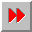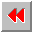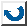EMA-XPS Online

DEFINE-CONSTRAINT-PATTERN

```DEFINE-CONSTRAINT-PATTERN
=========================

syntax: (define-constraint-pattern <name>
(<log-var>+)
[:DOCUMENTATION <doc-string>]
[:EXPLANATION <expl-expr.>]
RULES
(known (<log-var>+)
and-if <complex-predication>
then {:INCONSISTENT | <assign-form>+})

Warning: The explanation facility is not sup-
ported, hence :documentation and
:explanation are ignored.

The constraint-pattern forms a pattern for the
definition of the constraints. The defined content
of the constraint-pattern kann be worked out for
every single constraint.
The KNOWN-clause determines which of the logical-
variables have to be linked together, in order to
have a true rule. The if-condition is not obligatory,
the only conventions which are valid for it are those
of the BQL. In the premise-part of the rules logical
variables can be used in addition to the known para-
meters, but no unknown parameters must be used.
Only the first valid instance of the regulating
premise will be searched for.
If a behavior is :inconsistent, then the constraint
will be stopped.
The <assign-form> links a value to a variable, which
must not be declared as 'known'.
In addition to a constraint-pattern single constraints
have to be defined for which this behavior has to be
valid.

example: >(define-constraint-pattern
calculate-real-costs)
(_real-costs _country-wishes)
:documentation "calculates the
costs for a visit of the countries
_country-wishes."
RULES
(KNOWN (_country-wishes)
THEN (is _real-costs (calculate-client-
costs _country-wishes))))
>(define-constraint calculate-costs-for-
client
OF calculate-real-costs
FOR-EACH (a-client (instances-of
'client)))
WITH
[complete-costs a-client _real-costs]
[destinations a-client _country-wishes])

```EMA-XPS Online